The ARIMA Procedure

The ARIMA procedure primarily uses the computational methods outlined by Box and Jenkins. Marquardt’s method is used for the nonlinear least squares iterations. Numerical approximations of the derivatives of the sum-of-squares function are taken by using a fixed delta (controlled by the DELTA= option).

The methods do not always converge successfully for a given set of data, particularly if the starting values for the parameters are not close to the least squares estimates.

### Back-Forecasting

The unconditional sum of squares is computed exactly; thus, back-forecasting is not performed. Early versions of SAS/ETS software used the back-forecasting approximation and allowed a positive value of the BACKLIM= option to control the extent of the back-forecasting. In the current version, requesting a positive number of back-forecasting steps with the BACKLIM= option has no effect.

### Preliminary Estimation

If an autoregressive or moving-average operator is specified with no missing lags, preliminary estimates of the parameters are computed by using the autocorrelations computed in the IDENTIFY stage. Otherwise, the preliminary estimates are arbitrarily set to values that produce stable polynomials.

When preliminary estimation is not performed by PROC ARIMA, then initial values of the coefficients for any given autoregressive or moving-average factor are set to 0.1 if the degree of the polynomial associated with the factor is 9 or less. Otherwise, the coefficients are determined by expanding the polynomial (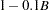) to an appropriate power by using a recursive algorithm.

These preliminary estimates are the starting values in an iterative algorithm to compute estimates of the parameters.

### Estimation Methods

#### Maximum Likelihood

The METHOD= ML option produces maximum likelihood estimates. The likelihood function is maximized via nonlinear least squares using Marquardt’s method. Maximum likelihood estimates are more expensive to compute than the conditional least squares estimates; however, they may be preferable in some cases (Ansley and Newbold 1980; Davidson 1981).

The maximum likelihood estimates are computed as follows. Let the univariate ARMA model be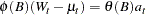where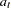is an independent sequence of normally distributed innovations with mean 0 and variance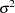. Here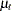is the mean parameter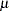plus the transfer function inputs. The log-likelihood function can be written as follows: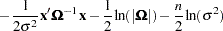In this equation, n is the number of observations,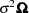is the variance of x as a function of the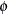and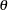parameters, and
denotes the determinant. The vector x is the time series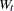minus the structural part of the model, written as a column vector, as follows: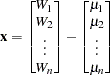The maximum likelihood estimate (MLE) ofis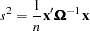Note that the default estimator of the variance divides by, where r is the number of parameters in the model, instead of by n. Specifying the NODF option causes a divisor of n to be used.

The log-likelihood concentrated with respect tocan be taken up to additive constants as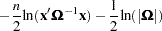Let H be the lower triangular matrix with positive elements on the diagonal such that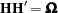. Let e be the vector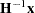. The concentrated log-likelihood with respect tocan now be written as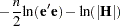or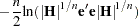The MLE is produced by using a Marquardt algorithm to minimize the following sum of squares: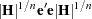The subsequent analysis of the residuals is done by using e as the vector of residuals.

#### Unconditional Least Squares

The METHOD=ULS option produces unconditional least squares estimates. The ULS method is also referred to as the exact least squares (ELS) method. For METHOD=ULS, the estimates minimize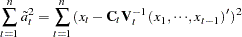where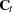is the covariance matrix of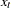and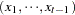, and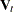is the variance matrix of. In fact,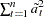is the same as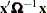, and hence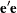. Therefore, the unconditional least squares estimates are obtained by minimizing the sum of squared residuals rather than using the log-likelihood as the criterion function.

#### Conditional Least Squares

The METHOD=CLS option produces conditional least squares estimates. The CLS estimates are conditional on the assumption that the past unobserved errors are equal to 0. The seriescan be represented in terms of the previous observations, as follows: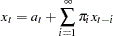The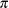weights are computed from the ratio of theandpolynomials, as follows: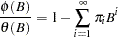The CLS method produces estimates minimizing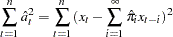where the unobserved past values ofare set to 0 and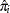are computed from the estimates ofandat each iteration.

For METHOD=ULS and METHOD=ML, initial estimates are computed using the METHOD=CLS algorithm.

### Start-up for Transfer Functions

When computing the noise series for transfer function and intervention models, the start-up for the transferred variable is done by assuming that past values of the input series are equal to the first value of the series. The estimates are then obtained by applying least squares or maximum likelihood to the noise series. Thus, for transfer function models, the ML option does not generate the full (multivariate ARMA) maximum likelihood estimates, but it uses only the univariate likelihood function applied to the noise series.

Because PROC ARIMA uses all of the available data for the input series to generate the noise series, other start-up options for the transferred series can be implemented by prefixing an observation to the beginning of the real data. For example, if you fit a transfer function model to the variable Y with the single input X, then you can employ a start-up using 0 for the past values by prefixing to the actual data an observation with a missing value for Y and a value of 0 for X.

### Information Criteria

PROC ARIMA computes and prints two information criteria, Akaike’s information criterion (AIC) (Akaike 1974; Harvey 1981) and Schwarz’s Bayesian criterion (SBC) (Schwarz 1978). The AIC and SBC are used to compare competing models fit to the same series. The model with the smaller information criteria is said to fit the data better. The AIC is computed as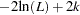where L is the likelihood function and k is the number of free parameters. The SBC is computed as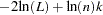where n is the number of residuals that can be computed for the time series. Sometimes Schwarz’s Bayesian criterion is called the Bayesian information criterion (BIC).

If METHOD=CLS is used to do the estimation, an approximation value of L is used, where L is based on the conditional sum of squares instead of the exact sum of squares, and a Jacobian factor is left out.

### Tests of Residuals

A table of test statistics for the hypothesis that the model residuals are white noise is printed as part of the ESTIMATE statement output. The chi-square statistics used in the test for lack of fit are computed using the Ljung-Box formula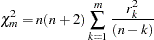where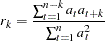andis the residual series.

This formula has been suggested by Ljung and Box (1978) as yielding a better fit to the asymptotic chi-square distribution than the Box-Pierce Q statistic. Some simulation studies of the finite sample properties of this statistic are given by Davies, Triggs, and Newbold (1977) and by Ljung and Box (1978). When the time series has missing values, Stoffer and Toloi (1992) suggest a modification of this test statistic that has improved distributional properties over the standard Ljung-Box formula given above. When the series contains missing values, this modified test statistic is used by default.

Each chi-square statistic is computed for all lags up to the indicated lag value and is not independent of the preceding chi-square values. The null hypotheses tested is that the current set of autocorrelations is white noise.

### t-values

The t values reported in the table of parameter estimates are approximations whose accuracy depends on the validity of the model, the nature of the model, and the length of the observed series. When the length of the observed series is short and the number of estimated parameters is large with respect to the series length, the t approximation is usually poor. Probability values that correspond to a t distribution should be interpreted carefully because they may be misleading.

### Cautions during Estimation

The ARIMA procedure uses a general nonlinear least squares estimation method that can yield problematic results if your data do not fit the model. Output should be examined carefully. The GRID option can be used to ensure the validity and quality of the results. Problems you might encounter include the following:

• Preliminary moving-average estimates might not converge. If this occurs, preliminary estimates are derived as described previously in Preliminary Estimation. You can supply your own preliminary estimates with the ESTIMATE statement options.

• The estimates can lead to an unstable time series process, which can cause extreme forecast values or overflows in the forecast.

• The Jacobian matrix of partial derivatives might be singular; usually, this happens because not all the parameters are identifiable. Removing some of the parameters or using a longer time series might help.

• The iterative process might not converge. PROC ARIMA’s estimation method stops after n iterations, where n is the value of the MAXITER= option. If an iteration does not improve the SSE, the Marquardt parameter is increased by a factor of ten until parameters that have a smaller SSE are obtained or until the limit value of the Marquardt parameter is exceeded.

• For METHOD=CLS, the estimates might converge but not to least squares estimates. The estimates might converge to a local minimum, the numerical calculations might be distorted by data whose sum-of-squares surface is not smooth, or the minimum might lie outside the region of invertibility or stationarity.

• If the data are differenced and a moving-average model is fit, the parameter estimates might try to converge exactly on the invertibility boundary. In this case, the standard error estimates that are based on derivatives might be inaccurate.Previous Page | Next Page | Top of Page